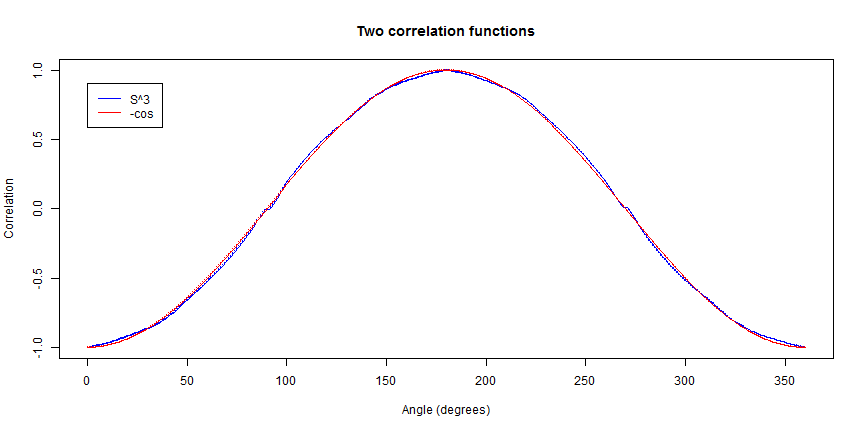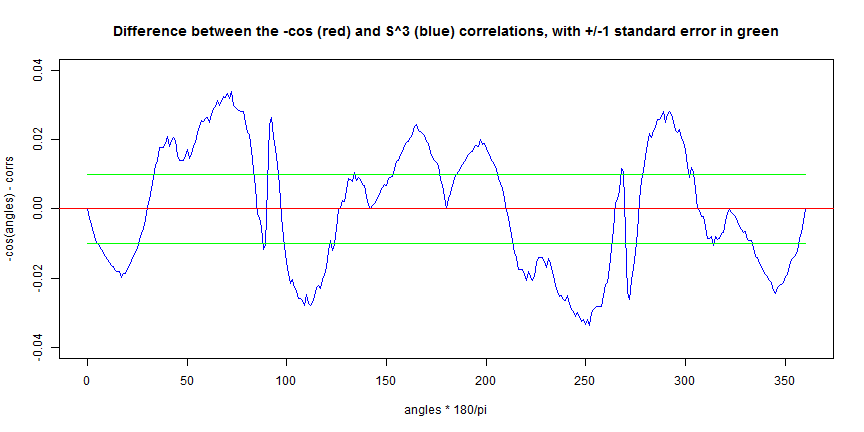My response to the 10,000 + 5,000 Euros challenge by Richard Gill:

``````## Richard Gill has offered 10,000 Euroes to anyone who can simulate the N
## directions of angular momentum vectors appearing in equation (16) of this
## experimental proposal of mine: http://arxiv.org/abs/0806.3078. Here I am
## attempting to provide such N directions. They are given by the vectors 'u'
## in this simulation. He has also offered further 5,000 Euros to me if my
## proposed experiment is realized successfully. I am hopeful that that will
## happen some day. The details of these challanges by Richard Gill can be
## found here: http://www.sciphysicsforums.com/spfbb1/viewtopic.php?f=6&t=46.
## While this is by no means a perfect simulation of my model, it does meet
## all the stringent conditions set out by Richard Gill for his challenge.

## Since after the explosion the angular momentum vectors 'u' moving along
## the z direction will be confined to the x-y plane, a 2D simulation is good
## enough for my proposed experiment.

set.seed(9875)

## Measurement angles for directions 'a' and 'b' in the equatorial plane

angles <- seq(from = 0, to = 360, by = 1) * 2 * pi/360

K <- length(angles)

corrs <- numeric(K)  ## Container for correlations

L <- numeric(K)

N <- 10^4  ## Sample size. Next try 10^5, or even 10^6

z <- runif(N, -1, 1)

p <- sqrt(1 - z^2)

r <- runif(N, 0, pi)

s <- runif(N, 0, pi)

t <- runif(N, -1, +1)

w <- runif(N, -1, +1)

x <- p * cos(r/0.69) * sign(t)

y <- p * 1.21 * (-1 + (2/(sqrt(1 + (3 * (0.963 * s)/pi))))) * sign(w)

u <- rbind(x, y, z)  ## A 3xN matrix. The N columns of u represent the
## x, y, and z coordinates of points on an approximate 2-sphere, S^2

for (i in 1:K) {
alpha <- angles[i]
a <- c(cos(alpha), sin(alpha), 0)  ## Measurement direction 'a'

for (j in 1:K) {
beta <- angles[j]
b <- c(cos(beta), sin(beta), 0)  ## Measurement direction 'b'

ua <- colSums(u * a)  ## Inner products of 'u' with 'a'
ub <- colSums(u * b)  ## Inner products of 'u' with 'b'

corrs[i] <- sum(sign(-ua) * sign(ub))/N

# corrs[i] <- sum(sign(-ua))/N
}
}
``````
``````plot(angles * 180/pi, corrs, type = "l", col = "blue", main = "Two correlation functions",
xlab = "Angle (degrees)", ylab = "Correlation")
points(angles * 180/pi, corrs, col = "blue", pch = ".", cex = 2)
lines(angles * 180/pi, -cos(angles), col = "red")
points(angles * 180/pi, -cos(angles), col = "red", pch = ".", cex = 1)

legend(x = 0, y = 0.9, legend = c("S^3", "-cos"), text.col = c("black", "black"),
lty = 1, col = c("blue", "red"))
````````````
for (i in 1:K) {
alpha <- angles[i]
a <- c(cos(alpha), sin(alpha), 0)  ## Measurement direction 'a'

for (j in 1:K) {
beta <- angles[j]
b <- c(cos(beta), sin(beta), 0)  ## Measurement direction 'b'

ua <- colSums(u * a)  ## Inner products of 'u' with 'a'
ub <- colSums(u * b)  ## Inner products of 'u' with 'b'

corrs[j] <- sum(sign(-ua) * sign(ub))/N

# corrs[j] <- sum(sign(-ua))/N

L[j] <- N

}
}
``````
``````plot(angles * 180/pi, corrs, type = "l", col = "blue", main = "Two correlation functions",
xlab = "Angle (degrees)", ylab = "Correlation")
points(angles * 180/pi, corrs, col = "blue", pch = ".", cex = 2)
lines(angles * 180/pi, -cos(angles), col = "red")
points(angles * 180/pi, -cos(angles), col = "red", pch = ".", cex = 1)

legend(x = 0, y = 0.9, legend = c("S^3", "-cos"), text.col = c("black", "black"),
lty = 1, col = c("blue", "red"))
````````````plot(angles * 180/pi, -cos(angles) - corrs, type = "l", col = "blue", ylim = c(-0.04,
+0.04), main = "Difference between the -cos (red) and S^3 (blue) correlations, with +/-1 standard error in green")
abline(h = 0, col = "red", lwd = 1)
lines(angles * 180/pi, 1/sqrt(L), col = "green")
lines(angles * 180/pi, -1/sqrt(L), col = "green")
``````